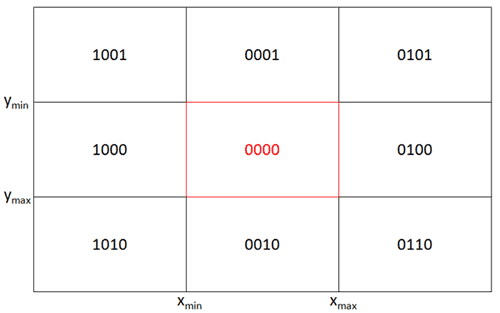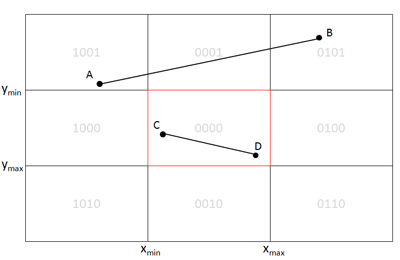2D Clipping with the Cohen-Sutherland-AlgorithmDownload Applet with Source (25kb)View Applet online

The Cohen-Sutherland-Algorithm is a well-known algorithm for clipping lines against a rectangle in 2D space. Although it is not the fastest one, the idea behind it is quite good and it works very well if a lot of lines lie outside completely outside the window/rectangle.

The main idea is following: Divide the complete 2D space in 9 areas where in the window to be clipped against is in the middle and the other 8 one are around it. To each of the nine regions a 4-bit code is assigned to identify it. Here a small picture to make it clear.For every endpoint of a line, we assign a region code to it depending on its position (x,y) (as convention we declare the leftmost bit as bit 3 and the rightmost bit 0) :

 Bit 3 is 1 <=> Point lies left from the window : x < xmin Bit 2 is 1 <=> Point lies right from the window : x > xmax Bit 1 is 1 <=> Point lies below from the window : y > ymax Bit 0 is 1 <=> Point lies above from the window : y < ymin

This idea allows us to handle two special cases easily. Therefore let's say we have a line from point p1 to point p2:

Case 1:
The line can be trivially rejected - that means it lies completely outside the window: code(p1) && code(p2) != 0.

Case 2:
The line can be trivially accepted - that means it lies completely inside the window: code(p1) || code(p2) == 0.

Example:Consider line AB: code (A) = 1001 code (B) = 0101 => 1001 && 0101 = 0001 != 0 => completely outside!   Consider line CD: code(A) = 0000 code(B) = 0000 => 0000 || 0000 = 0000 => completely visible (can be drawn immediately)

In all other cases, if the line cannot be trivially rejected or accepted, it has to be intersected. Therefore the intersection with the four window edges is calculated step by step, the line endpoints are updated with these new ones and the trivial cases are tested again after each intersection. The intersection itself is quite easy cause the four window edges are the two horizontal lines ymax and ymin and the two vertical ones xmax and xmin. So can calculate the intersection point with some thinking and linear interpolation (see my source if you are more interested in).

So here some pseudcode:

 1. Compute the region codes for both endpoints of the line. 2. if bitwise or is zero, the line lies completely inside the window and can be drawn without further calculation. 3. if bitwise and is not zero, the line lies completely outside the window and can be skipped. 4. At this point, at least one endpoint is outside the window, so check it's code and intersect it with the corresponding window edge. 5. Update the old endpoint with the new calculated one from step 4) and repeat the algorithm.

Example:Consider line AB: code(A) = 0001, code(B) = 0110 => both trivial cases not met look at Point A: bit 0 is set, so it's above the window => intersect it with ymin, we get point A1 repeat, our new line is A1B: code(A1) = 0000, code(B) = 0110 => both trivial cases not met look at Point B: bit 1 is set, so it's below the window => intersect it with ymax, we get point B1 repeat, our new line is A1B1: code(A1) = 0000, code(B1) = 0100 => both trivial cases not met look at Point B1: bit 2 is set, so it's right the window => intersect it with xmax, we get Point B2 repeat, our new line is A1B2: code(A1) = 0000, code(B2) = 0000 => Bitwise OR = 0 => line can now be drawn, completely visible

Ok, so have fun with it :-)

This site is part of Sunshine's Homepage